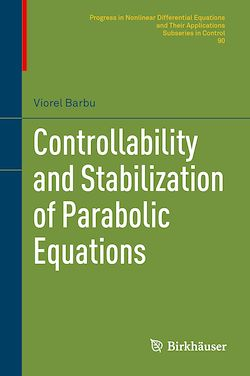226 Pages
English

# Controllability and Stabilization of Parabolic Equations

-Description

This monograph presents controllability and stabilization methods in control theory that solve parabolic boundary value problems. Starting from foundational questions on Carleman inequalities for linear parabolic equations, the author addresses the controllability of parabolic equations on a variety of domains and the spectral decomposition technique for representing them. This method is, in fact, designed for use in a wider class of parabolic systems that include the heat and diffusion equations. Later chapters develop another process that employs stabilizing feedback controllers with a finite number of unstable modes, with special attention given to its use in the boundary stabilization of Navier–Stokes equations for the motion of viscous fluid. In turn, these applied methods are used to explore related topics like the exact controllability of stochastic parabolic equations with linear multiplicative noise.

Intended for graduate students and researchers working on control problems involving nonlinear differential equations, Controllability and Stabilization of Parabolic Equations is the distillation of years of lectures and research. With a minimum of preliminaries, the book leaps into its applications for control theory with both concrete examples and accessible solutions to problems in stabilization and controllability that are still areas of current research.

Subjects

##### Technology

Informations